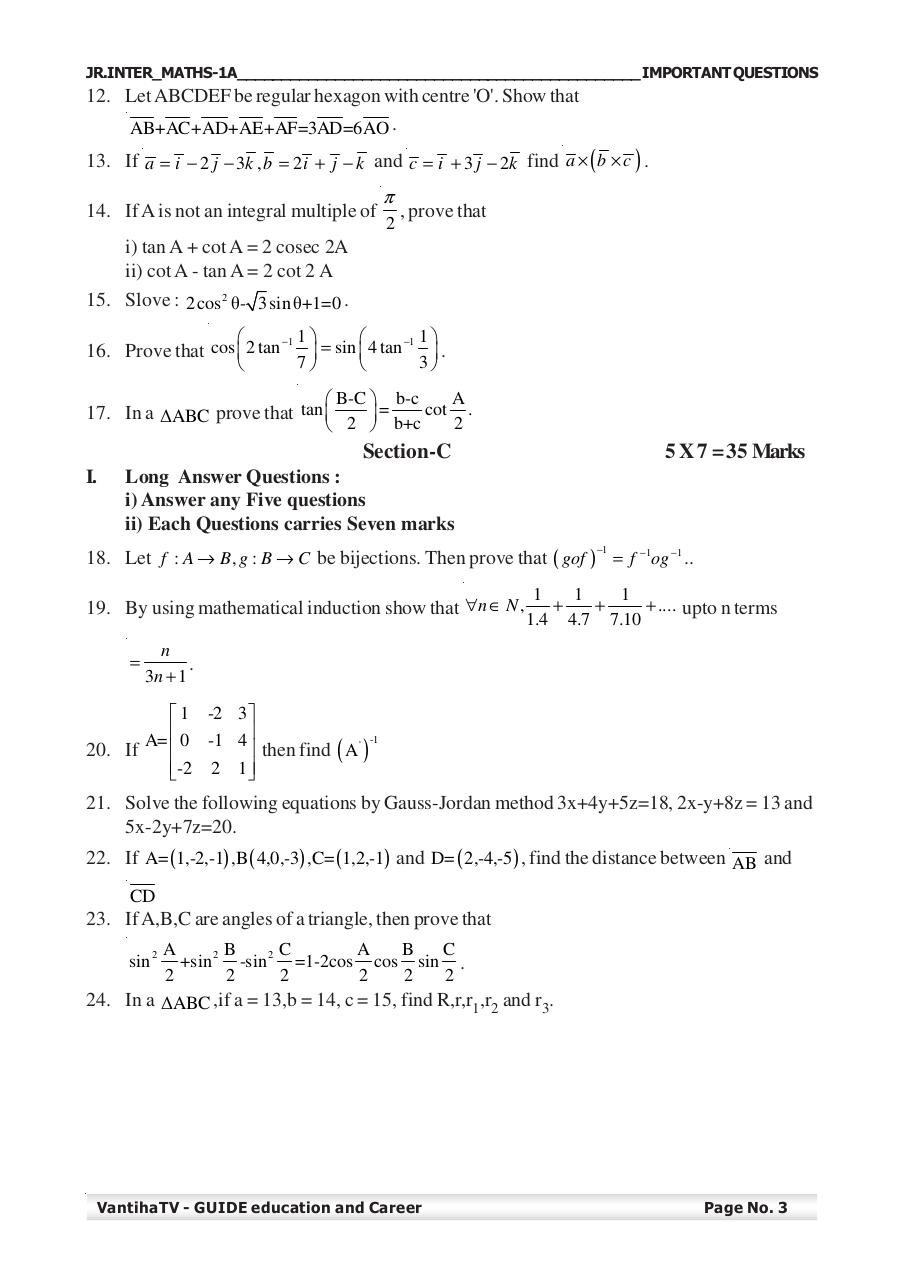# Maths 1A Chapter wise important Questions.pdfPage 1 2 34527

#### Text preview

JR.INTER_MATHS-1A_____________________________________________ IMPORTANT QUESTIONS

12. Let ABCDEF be regular hexagon with centre 'O'. Show that
AB+AC+AD+AE+AF=3AD=6AO .
13. If a = i − 2 j − 3k , b = 2 i + j − k and c = i + 3 j − 2k find a × ( b × c ) .
14. If A is not an integral multiple of

π
2

, prove that

i) tan A + cot A = 2 cosec 2A
ii) cot A - tan A = 2 cot 2 A
15. Slove : 2 cos 2 θ- 3 sin θ+1=0 .

1

1

−1
−1
16. Prove that cos  2 tan
 = sin  4 tan
.
7
3

A
 B-C  b-c
cot .
17. In a ∆ABC prove that tan 
=
2
 2  b+c

Section-C
I.

5 X 7 = 35 Marks

Long Answer Questions :
i) Answer any Five questions
ii) Each Questions carries Seven marks
−1

18. Let f : A → B, g : B → C be bijections. Then prove that ( gof ) = f −1og −1 ..
19. By using mathematical induction show that ∀n ∈ N ,
=

1
1
1
+
+
+ .... upto n terms
1.4 4.7 7.10

n
.
3n + 1

 1 -2 3

-1
20. If A=  0 -1 4 then find ( A ' )
-2 2 1

21. Solve the following equations by Gauss-Jordan method 3x+4y+5z=18, 2x-y+8z = 13 and
5x-2y+7z=20.
22. If A= (1,-2,-1) ,B ( 4,0,-3) ,C= (1,2,-1) and D= ( 2,-4,-5) , find the distance between AB and
CD

23. If A,B,C are angles of a triangle, then prove that
sin 2

A
B
C
A
B
C
+sin 2 -sin 2 =1-2cos cos sin .
2
2
2
2
2
2

24. In a ∆ABC ,if a = 13,b = 14, c = 15, find R,r,r1,r2 and r3.

VantihaTV - GUIDE education and Career

Page No. 3